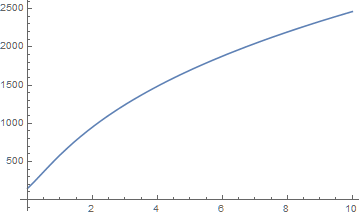# How do I calculate mortgages and loans using annuities?

The TimeValue expression can describe the present value of an Annuity in terms of the cost of each payment, the number of periods, the size of the intervals, and the interest rate.

``` TimeValue[Annuity[payments, periods, intervals], interest, 0] ```

To find the monthly payments on a mortgage with value, equate the present value with some value:

``` TimeValue[Annuity[payments, periods, intervals], interest, 0] == value ```

Use the function Solve to solve the equation in terms of payments:

``` Solve[TimeValue[Annuity[payments, periods, intervals], interest, 0] == value, payments] ```

This returns an algebraic answer for the value of the payments in terms of the other variables. Solve outputs the result as a list of rules.

Solve can calculate with numbers as well. For example, to calculate the payments on a \$200,000 mortgage amortized over 30 years at 5.2% nominal interest, evaluate:

``` Solve[TimeValue[Annuity[payments, 30, 1/12], EffectiveInterest[.052, 1/12], 0] == 200000, payments] ```

```{{payments -> 1098.22}} ```

We can solve for other parameters as well, such as the number of payments. To find the number of years required to pay off a \$10,000 loan with payments of \$200 per month at an 8% interest rate, evaluate:

``` Solve[TimeValue[Annuity[200,periods, 1/12], .08, 0] == 10000,periods] ```

```{{periods -> 5.04369}} ```

To calculate the monthly payments needed to pay off a \$5,000 loan in 3 years, evaluate:

``` Solve[TimeValue[Annuity[payments, 3, 1/12], .08, 0] == 5000, payments] ```

```{{payments -> 156.039}} ```

A loan of \$3,000 is repaid with quarterly installments at the end of each quarter for 5 years. If the interest rate is 10% compounded semiannually, the amount of each quarterly payment is found with the following code:

``` Solve[TimeValue[Annuity[payments, 5, 1/4], EffectiveInterest[.1, 1/2], 0] == 3000, payments] ```

```{{payments -> 191.888}} ```

Solve can also solve for the relationship between two parameters. For example we can find the function relating payments to the interest rate and then plot the relationship:

``` relationship = First[Solve[ TimeValue[Annuity[payments, 5, 1/4], interest, 0] == 3000, payments]]```

``` ```

```Plot[payments /. relationship, {interest, 0, 10}] ```Este artigo foi útil?

Algum comentário?

## Entre em contato com o Suporte

Caso tenha alguma dúvida sobre cobrança, ativação ou suporte técnico, estamos a sua total disposição.Ligue para a Wolfram

## Suporte ao consumidor

Segunda–Sexta
8:00–17:00 Horário Central dos EUA

• Registro ou ativação do produto
• Informações de pré-venda e pedidos
• Ajuda com instalação e início